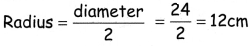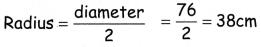Students can download 4th Maths Term 1 Chapter 1 Geometry Ex 1.4 Questions and Answers, Notes, Samacheer Kalvi 4th Maths Guide Pdf helps you to revise the complete Tamilnadu State Board New Syllabus, helps students complete homework assignments and to score high marks in board exams.

## Tamilnadu Samacheer Kalvi 4th Maths Solutions Term 1 Chapter 1 Geometry Ex 1.4

A. Fill in the blanks.

(i) All the radii of a circle are ________ .
equal

(ii) The ________ is the longest chord of a circle.
diameter(iii) A line segment joining any point on the circle to its center is called ________ the of the circle.

(iv) A line segment with its end points on the circle is called a ________.
chord

(v) Twice the radius is ________.
diameterB. Find the diameter of the circle

Diameter = 2 × radius = 2 × 10
Diameter = 20 cm

Diameter = 2 × radius = 2 × 8
Diameter = 16 cm

Diameter = 2 × radius = 2 × 6
Diameter = 12 cmC. Find the radius of the circle.

(i) Diameter = 24 cm
(ii) Diameter = 30 cm
(iii) Diameter = 76 cm